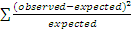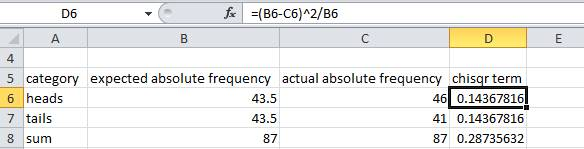# BSCI 1511L Statistics Manual: 1 Probabilities, frequencies, and the Chi Squared Goodness of Fit test

Introduction to Biological Sciences lab, second semester

## Learning Objectives

At the end of this section, you should be able to:

• use the following pairs of terms appropriately: continuous vs. discontinuous, expected vs. actual (or observed). and relative vs. absolute
• describe the relationship between probability and relative frequency
• convert between relative and absolute frequencies when the total count is known
• describe the type of data that can be analyzed in a chi squared goodness of fit test, and the appropriate null and alternative hypotheses
• know the formula for calculating a chi squared value
• perform a chi-squared goodness of fit test using Excel
• estimate probability using empirically determined counts of outcomes

## 1.1 Relative frequency and probability

1.1 Relative frequency and probability

If one flips a normal coin, it is equally likely that one will obtain heads or tails.  One way of expressing this is to say that the ratio of heads to tails is 1:1 .   Another way of expressing the relationship is to describe the relative frequency of each outcome.  The relative frequency is the fraction of times each outcome is achieved.  Relative frequency can be calculated by taking the count of an individual kind of outcome and divide by the total counts for all kinds of outcomes.  For a ratio of 1:1, there are two total outcomes, so the relative frequency of heads is ½ or 0.5 and the relative frequency of tails is the same.  It is normal practice to express relative frequencies as decimal fractions.

One can also express this relationship using probability.  If a system behaves consistently over time, it is reasonable to expect that the relative frequency at which we observe a certain event is related to the probability of occurrence of that event.  If the probability of obtaining heads is 0.5, then if we flip a coin many times we would expect to obtain heads with a relative frequency of 0.5 .  Based on this assumption, we can state that the expected relative frequency of an outcome is equal to the probability of that outcome.  Based on the 1:1 ratio of heads to tails, the probability of obtaining tails is also 0.5 and the expected relative frequency is 0.5 as well.

Note that the two probabilities add up to one, which makes sense since the only possible outcomes are heads and tails.  The sum of relative frequencies is also equal to one, since the sum of all fractional parts must equal the whole.

These ideas are summarized in Table 1:

Table 1. Relationship between ratio, frequency, and probability for a penny

 normal penny ratio fraction expected relative frequency (decimal) probability of outcome heads 1 1/2 0.5 0.5 tails 1 1/2 0.5 0.5

## 1.2 Absolute and relative frequencies

Assume that you have a normal penny.  You flip it 87 times and get heads 46 times and tails 41 times.  We can describe these outcomes in terms of absolute frequencies.  The absolute frequency is the actual number of times an outcome is observed.  In this case we would say that the absolute frequencies are 46 heads and 41 tails.

Absolute frequency can be converted to relative frequency by finding the fraction of the time that outcome occurs.  We can summarize the situation as follows:

Table 2. Relationship between absolute and relative frequency for some penny flips

 normal penny actual absolute frequency fraction actual relative frequency heads 46 46/87 0.529 tails 41 41/87 0.471

In Table 2, the frequencies are described as "actual" because they are outcomes we actually observed.  They are also sometimes referred to as "observed absolute frequency" and "observed relative frequency".

## 1.3 Deviation from expected frequencies

The outcomes recorded in Table 2 are not exactly what we expected because we expect that a normal coin should produce equal numbers of heads and tails.  Because the probability of an outcome determines the expected relative frequency, we expect relative frequencies of 0.5 heads and 0.5 tails for a normal penny.  It is easy to calculate the expected absolute frequencies by multiplying the expected relative frequencies (=probabilities) by the total number of outcomes.  For a total of 87 coin flips, we expect (0.5)(87)=43.5 heads.  Since the probability of tails is the same, we also expect 43.5 tails.  We should not be concerned that the expected absolute frequency includes a fraction and we should not round it, even though we know that in reality the number of outcomes must be a whole number.  The situation is summarized in Table 3.

Table 3. Comparison of expected and actual frequencies for a normal penny

 normal penny expected relative frequency/probability expected absolute frequency actual absolute frequency actual relative frequency heads 0.5 43.5 46 0.529 tails 0.5 43.5 41 0.471

## 1.4 Assessing deviations from expected frequencies using the Chi Squared Goodness of Fit test

The outcomes shown in Table 3 might cause us to expect that the coin was loaded because there were more heads than we expected.  However, as we learned last semester, it is common to observe small deviations from what we expect due to chance variation.  So we should not be surprised to observe an outcome of 46 heads and 41 tails even if the coin were not loaded.  If the deviation from the expected frequency were a lot greater (for example 61 heads and 26 tails), we might suspect that something unusual was going on.

We can assess the significance of the deviation from expected by calculating a statistic called chi squared (χ2).  The chi squared statistic is calculated using the following formula:

χ2 =where for each term in the summation "observed" is the actual absolute frequency for a category and "expected" is the expected absolute frequency for the same category.  The terms for all categories are summed to create the overall chi squared value.  Note: you must use counts in this formula!  You cannot use relative frequencies!

You can see from this formula that the squaring the deviation of a category from its expected value causes large deviations to have a much greater effect than small ones.  In the case of the example in Table 3, the value of chi squared would be:

(46-43.5)2/43.5+(41-43.5)2/43.5=0.287

In the case where 61 heads and 26 tails were flipped, the value of chi squared would be 14.080 .  Greater deviations would result in even greater chi squared values.

The calculated chi squared value can be used to assess the significance of the deviation from the expected frequencies as part of a test known as a chi squared goodness of fit test.  In a goodness of fit test, the null hypothesis is that there is no deviation from the expected frequencies.  The alternative hypothesis is that the actual (observed) frequencies differ from the expected frequencies.

In the goodness of fit test, the number of degrees of freedom is related to the number of categories.  For a simple list of categories, the number of degrees of freedom is the number of categories minus one.  So in the coin flip example, the number of degrees of freedom is 2-1=1.

For a particular number of degrees of freedom, there is an inverse relationship between the chi squared value and P, the probability that the deviation from the expected frequencies is due only to chance.  As with all of the other statistical tests we have used, when the value of the statistic is great enough to cause P to be less than 0.05, we say that there is a significant difference between the expected frequencies and the actual observed frequencies.

## 1.5 Performing a chi squared goodness of fit test using Excel

Step 1. Enter the absolute frequencies. Create columns for the expected absolute frequencies and actual absolute frequencies.

Fig. 1. Calculation of chi squared goodness of fit terms using ExcelStep 2. Create a formula for a chi squared term. In an adjacent column, create a formula for the chi squared term for that category (column D in Fig. 1).  Excel uses normal order of operations, so use parentheses to force the subtraction before exponentiation.  The squaring will be done before the division, which is OK.

Step 3. Calculate all of the chi squared terms.  Use the fill handle (or copy and paste) to copy the formula into the rows for the other categories.

Step 4. Calculate the total chi squared value. Use the sum function to calculate the total of the first frequency column.  Then copy that formula across to the other columns, including the column for the chi squared terms.  The sum of the chi squared terms is the value of the chi squared statistic for the test.

Step 5. Calculate the P-value.  To calculate the value of P, click on a new cell, then select the CHISQ.TEST function from the list of statistical functions.  In the box for “Actual_range”, enter the range of cells that contain the actual absolute frequencies (do NOT include the sum).  In the box for “Expected_range”, enter the range of cells that contain the expected absolute frequencies.  In the example above, the resulting formula is =CHISQ.TEST(C6:C7,B6:B7) and the formula produces a P-value of 0.592 which indicates that the actual absolute frequency does not deviate significantly from the expected.  Test the spreadsheet, by replacing the actual frequencies of 46 and 41 with the more extreme values of 61 and 26.  The chi squared value should change to 14.080 and the P-value to 0.000175 which shows that these frequencies deviate significantly from the expected.

## 1.6 Assessing probability empirically

The coin flip example described above assumes a priori knowledge about the probabilities of flip outcomes for normal coins.  In biology, we often do not know in advance the probability of certain outcomes.  Instead, we predict outcomes in the future by assuming that systems will behave in the same way that they did in the past.  We can measure a large number of outcomes and use that information to infer probabilities that can be used to predict future outcomes.

Imagine that a magician friend gives you a nickel and tells you that it is loaded to produce tails more often than heads.  If your friend does not tell you the probability of achieving heads and tails, you will have to determine that empirically by flipping the coin many times and recording the outcomes.  Some example results are shown in Table 4

Table 4. Empirically derived frequencies for a loaded coin

 trick nickel actual absolute frequency relative frequency heads 394 0.311 tails 873 0.689 total 1267 1.000

As in Table 2, the relative frequency is a decimal fraction calculated by dividing the absolute frequency of one category by the total count.

If we wanted to predict the likelihood of achieving heads at some point in the future using the trick nickel, we can assume that the relative frequency that we calculated represents the probability of that outcome.  In this case, we would say that the probability of heads would be P=0.311 and the probability of tails would be P=0.689 .

In summary, we assume that the relative frequency of an event observed in the past represents the probability of that event occurring in the future.